Home Machine Learning Machine Learning Education Basics of Deep Learning and Neural Networks

# Basics of Deep Learning and Neural Networks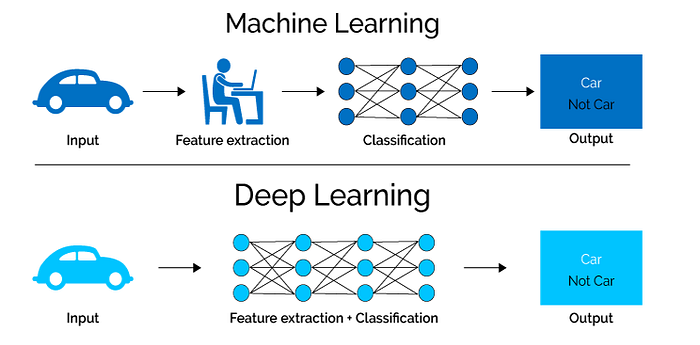Feature Extraction is only required for ML Algorithms.

#### In other words, we can also say that the feature extraction step is already part of the process that takes place in an artificial neural network.

During the training process, this step is also optimized by the neural network to obtain the best possible abstract representation of the input data. This means that the models of deep learning thus require little to no manual effort to perform and optimize the feature extraction process.

Let us look at a concrete example. For example, if you want to use a machine learning model to determine if a particular image is showing a car or not, we humans first need to identify the unique features or features of a car (shape, size, windows, wheels, etc.) extract the feature and give them to the algorithm as input data.

In this way, the algorithm would perform a classification of the images. That is, in machine learning, a programmer must intervene directly in the action for the model to come to a conclusion.

In the case of a deep learning model, the feature extraction step is completely unnecessary. The model would recognize these unique characteristics of a car and make correct predictions.

That completely without the help of a human.

In fact, refraining from extracting the characteristics of data applies to every other task you’ll ever do with neural networks. Just give the raw data to the neural network, the rest is done by the model.

## The Era of Big Data…

The second huge advantage of Deep Learning and a key part in understanding why it’s becoming so popular is that it’s powered by massive amounts of data. The “Big Data Era” of technology will provide huge amounts of opportunities for new innovations in deep learning. As per Andrew Ng, the chief scientist of China’s major search engine Baidu and one of the leaders of the Google Brain Project,

The analogy to deep learning is that the rocket engine is the deep learning models and the fuel is the huge amounts of data we can feed to these algorithms.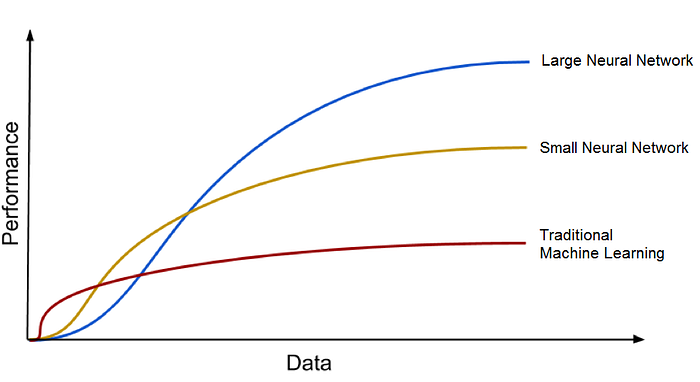Deep Learning Algorithms get better with the increasing amount of data.

Deep Learning models tend to increase their accuracy with the increasing amount of training data, where’s traditional machine learning models such as SVM and Naive Bayes classifier stop improving after a saturation point.

# 3. Biological Neural Networks

Before we move any further with artificial neural networks I would like to introduce the concept behind biological neural networks, so when we will later discuss the artificial neural network in more detail we can see parallels with the biological model.

Artificial neural networks are inspired by the biological neurons that are found in our brains. In fact, the artificial neural networks simulate some basic functionalities of the neural networks in our brain, but in a very simplified way. Let’s first look at the biological neural networks to derive parallels to artificial neural networks. In short, a biological neural network consists of numerous neurons.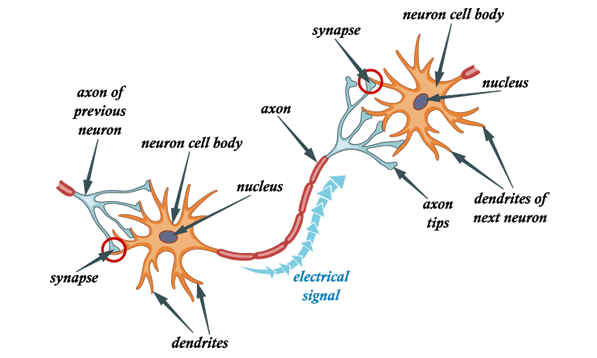A Model of a biological Neural Network.

A typical neuron consists of a cell body, dendrites, and an axon. Dendrites are thin structures that emerge from the cell body. An axon is a cellular extension that emerges from this cell body. Most neurons receive signals through the dendrites and send out signals along the axon.

At the majority of synapses, signals cross from the axon of one neuron to the dendrite of another. All neurons are electrically excitable due to the maintenance of voltage gradients in their membranes. If the voltage changes by a large enough amount over a short interval, the neuron generates an electrochemical pulse called an action potential. This potential travels rapidly along the axon and activates synaptic connections as it reaches them.

# 4. Artificial Neural Networks

Now that we have a basic understanding of how biological neural networks are functioning, let’s finally take a look at the architecture of the artificial neural network.

A neural network generally consists of a collection of connected units or nodes. We call these nodes neurons. These artificial neurons loosely model the biological neurons of our brain.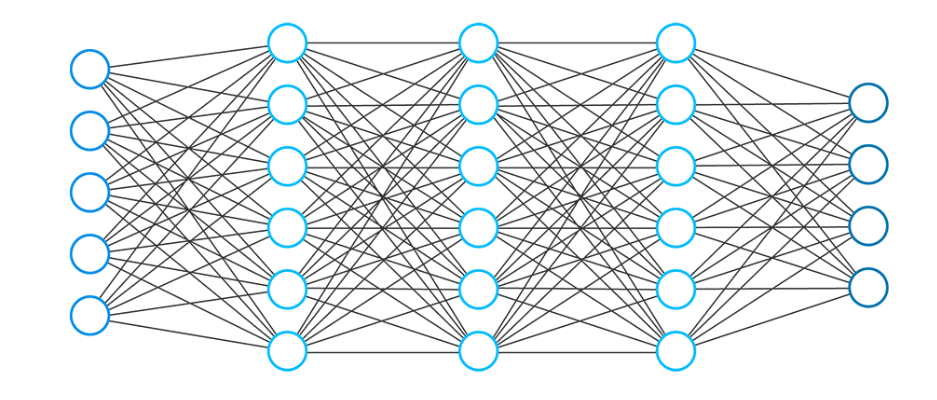An artificial Feedforward Neural Network.

A neuron is simply a graphical representation of a numeric value (e.g. 1.2, 5.0, 42.0, 0.25, etc.). Any connection between two artificial neurons can be considered as an axon in a real biological brain.

The connections between the neurons are realized by so-called weights, which are also nothing more than numerical values

When an artificial neural network learns, the weights between neurons are changing and so does the strength of the connection Meaning: Given training data and a particular task such as classification of numbers, we are looking for certain set weights that allow the neural network to perform the classification. The set of weights is different for every task and every dataset. We can not predict the values of these weights in advance, but the neural network has to learn them. The process of learning we also call as training.

# 5. Typical Neural Network Architecture

The typical neural network architecture consists of several layers. We call the first layer as the input layer.

The input layer receives the input x, data from which the neural network learns. In our previous example of classification of handwritten numbers, these input x would represent the images of these numbers ( x is basically an entire vector where each entry is a pixel).

The input layer has the same number of neurons as there are entries in the vector x. Meaning: each input neuron represents one element in the vector x.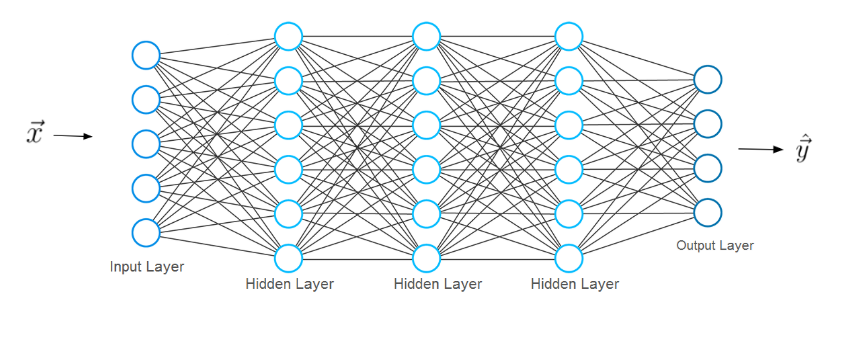Structure of a Feedforward Neural Network.

The last layer is called the output layer, which outputs a vector y representing the result that the neural network came up with. The entries in this vector represent the values of the neurons in the output layer. In our case of classification, each neuron in the last layer would represent a different class.

In this case, the value of an output neuron gives the probability that the handwritten digit given by the features x belongs to one of the possible classes (one of the digits 0–9). As you can imagine the number of output neurons must be the same as there are classes.

In order to obtain a prediction vector y, the network must perform certain mathematical operations. These operations are performed in the layers between the input and output layers. We call these layers the hidden layers. Now lets us discuss how the connections between the layers look like.

# 6. Layer Connections in a Neural Network

Please consider a smaller example of a neural network that consists of only two layers. The input layer has two input neurons, while the output layer consists of three neurons.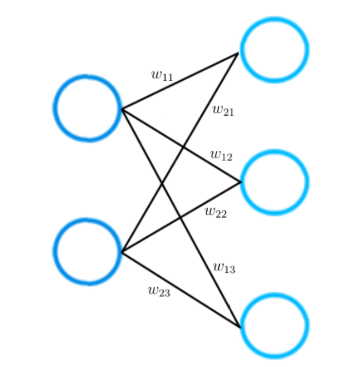Layer Connections

As mentioned earlier: each connection between two neurons is represented by a numerical value, which we call weight.

As you can see in the picture, each connection between two neurons is represented by a different weight w. Each of these weight w has indices. The first value of the indices stands for the number of neurons in the layer from which the connection originates, the second value for the number of the neurons in the layer to which the connection leads.

All weights between two neural network layers can be represented by a matrix called the weight matrix.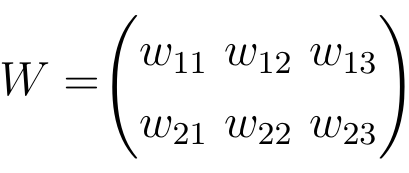A weight matrix.

A weight matrix has the same number of entries as there are connections between neurons. The dimensions of a weight matrix result from the sizes of the two layers that are connected by this weight matrix.

The number of rows corresponds to the number of neurons in the layer from which the connections originate and the number of columns corresponds to the number of neurons in the layer to which the connections lead.

In this particular example, the number of rows of the weight matrix corresponds to the size of the input layer which is two and the number of columns to the size of the output layer which is three.

# 8. Loss Functions

After we get the prediction of the neural network, in the second step we must compare this prediction vector to the actual ground truth label. We call the ground truth label as vector y_hat.

While the vector y contains the predictions that the neural network has computed during the forward propagation (and which may, in fact, be very different from the actual values), the vector y_hat contains the actual values.

Mathematically, we can measure the difference between and y_hat by defining a loss function which value depends on this difference.

An example of a general loss function is the quadratic loss: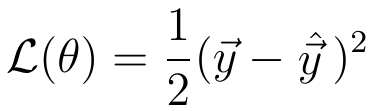Quadratic Loss.

The value of this loss function depends on the difference between y_hat and y. A higher difference means a higher loss value, a smaller difference means a smaller loss value.

Minimizing the loss function directly leads to more accurate predictions of the neural network, as the difference between the prediction and the label decreases.

Minimizing the loss function automatically causes the neural network model to make better predictions regardless of the exact characteristics of the task at hand. You only have to select the right loss function for the task. Fortunately, there are only two loss functions that you should know about to solve almost any problem that you encounter in practice.

These loss-functions are the Cross-Entropy Loss: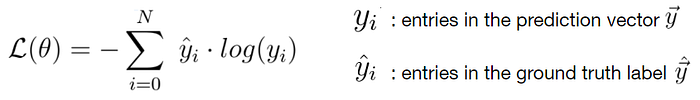Cross-Entropy Loss Function.

and the Mean Squared Error Loss: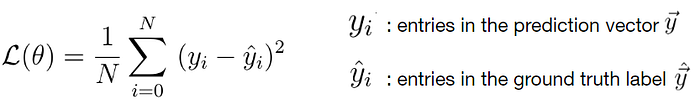Mean Squared Error Loss Function.

Since the loss depends on the weights, we must find a certain set of weights for which the value of the loss function is as small as possible. The method of minimizing the loss function is achieved mathematically by a method called gradient descent

# 9. Gradient Descent

During gradient descent, we use the gradient of a loss function (or in other words the derivative of the loss function) to improve the weights of a neural network.

To understand the basic concept of the gradient descent process, let us consider a very basic example of a neural network consisting of only one input and one output neuron connected by a weight value w.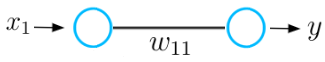Simple Neural Network.

This neural network receives an input x and outputs a prediction y. Let say the initial weight value of this neural network is 5 and the input x is 2. Therefore the prediction y of this network has a value of 10, while the label y_hat might have a value of 6.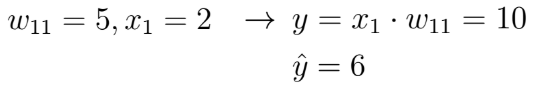Parameters and Predictions.

This means that the prediction is not accurate and we must use the gradient descent method to find a new weight value that causes the neural network to make the correct prediction. In the first step, we must choose a loss function for the task. Let’s take the quadratic loss that I have defined earlier and plot this function, which basically is just a quadratic function: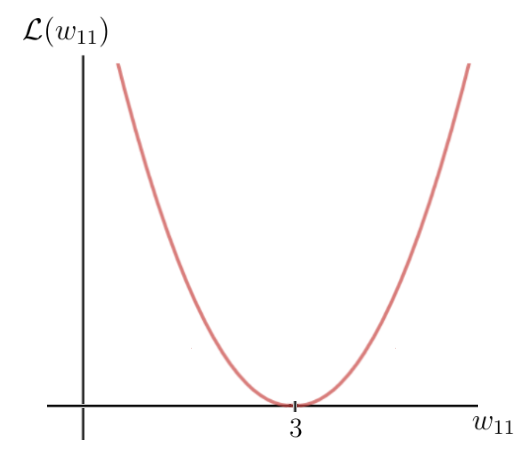Quadratic Loss Function.

The y-axis is the loss value which depends on the difference between the label and the prediction, and thus the network parameters, in this case, the one weight w. The x-axis represents the values for this weight. As you can see there is a certain weight w for which the loss function reaches a global minimum. This value is the optimal weight parameter that would cause the neural network to make the correct prediction which is 6. In this case, the value for the optimal weight would be 3: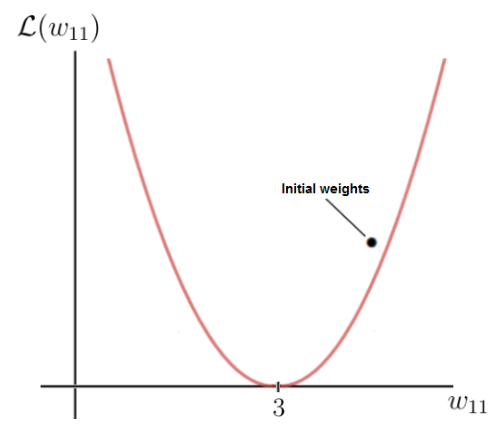Initial Weight Value.

Our initial weight, on the other hand, is 5, which leads to a fairly high loss. The goal now is to repeatedly update the weight parameter until we reach the optimal value for that particular weight. This is the time when we need to use the gradient of the loss function. Fortunately, in this case, the loss function is a function of one single variable, which is the weight w: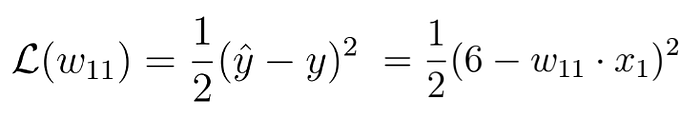Loss Function.

In the next step, we calculate the derivative of the loss function with respect to this parameter: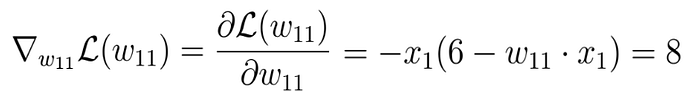Gradient of the loss function.

In the end, we get a result of 8, which gives us the value of the slope or the tangent of the loss function for the corresponding point on the x-axis at which our initial weight lies.

This tangent points towards the highest rate of increase of the loss function and the corresponding weight parameters on the x-axis.

This means that we have just used the gradient of the loss function to find out which weight parameters would result in an even higher loss value. But what we want to know is the exact opposite. We can get what we want, if we multiply the gradient by minus 1 and this way obtain the opposite direction of the gradient. This way we get the direction of the highest rate of decrease of the loss function and the corresponding parameters on the x-axis that cause this decrease:

In the final step, we perform one gradient descent step as an attempt to improve our wights. We use this negative gradient to update your current weight in the direction of the weights for which the value of the loss function decreases according to the negative gradient: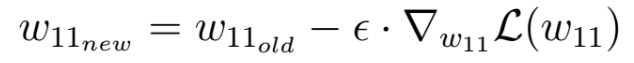Gradient Descent Step.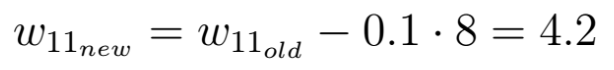Gradient Descent Step.

The factor epsilon in this equation is a hyperparameter called the learning rate. The learning rate determines how quickly or how slowly you want to update the parameters. Please keep in mind that the learning rate is the factor with which we have to multiply the negative gradient and that the learning rate is usually quite small. In our case, the learning rate is 0.1.

As you can see, our weight w after the gradient descent is now 4.2 and closer to the optimal weight than it was before the gradient step.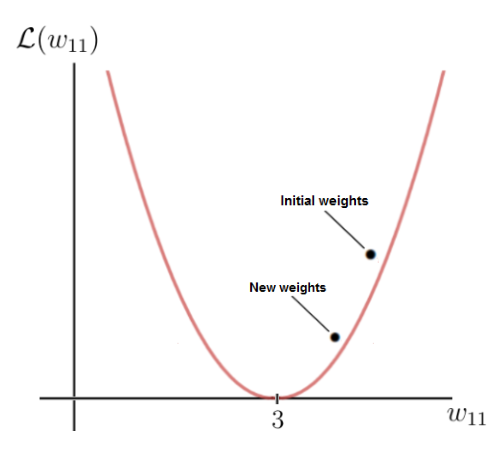New Weights after Gradient Descent.

The value of the loss function for the new weight value is also smaller, which means that the neural network is now capable to do a better prediction. You can do the calculation in your head and see that the new prediction is, in fact, closer to the label than before.

Each time we are performing the update of the weights, we move down the negative gradient towards the optimal weights.

After each gradient descent step or weight update, the current weights of the network get closer and closer to the optimal weights until we eventually reach them and the neural network will be capable to do the predictions we want to make.

This article has been published from the source link without modifications to the text. Only the headline has been changed.

- Advertisment -### Lockdowns’ benefit on Data Centre buildings

- Advertisment -